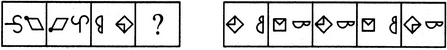# Non Verbal Reasoning - Analogy - Discussion

### Discussion :: Analogy - Section 1 (Q.No.43)

Each of the following questions consists of two sets of figures. Figures A, B, C and D constitute the Problem Set while figures 1, 2, 3, 4 and 5 constitute the Answer Set. There is a definite relationship between figures A and B. Establish a similar relationship between figures C and D by selecting a suitable figure from the Answer Set that would replace the question mark (?) in fig. (D).

43.

Select a suitable figure from the Answer Figures that would replace the question mark (?).(A)     (B)      (C)     (D)                  (1)      (2)      (3)      (4)      (5)

 [A]. 1 [B]. 2 [C]. 3 [D]. 4 [E]. 5

Explanation:

The two elements interchange positions; the element that reaches the LHS position gets vertically inverted and the element that reaches the RHS position, rotates 90oACW.

 Kaushik Chatterjee said: (Jan 8, 2015) Answer should be E. As RHS rotates 90 degree ACW.

 Raju Singh said: (Apr 24, 2015) I think answer is E. Because when right figure change 90° acw then it look like figure E.

 Ramesh said: (Aug 13, 2016) I think the answer is E.

 Vamsi said: (Sep 15, 2016) I think the answer should be E.

 Clare said: (Dec 1, 2016) According to given solution, i.e if rotated to 90 degree ACW then option should be option E.

 Ansab said: (Jul 7, 2017) Yes, E is correct. I also agree.

 Himanshu said: (Jul 10, 2017) Yes, I also think it's E.

 Arjun said: (Sep 2, 2017) I also agree, the correct answer must be E.

 Yuvraj said: (Feb 11, 2018) Yes, I also agree with E.

 Tushendra said: (Nov 25, 2018) E must be the answer because in C option it is rotating 90' CW but in E it rotates 90' ACW.

 Ajay Chhetry said: (Mar 29, 2019) Answer E is correct.

 Ramesh said: (Apr 4, 2019) The answer should be E.

 Saurabh said: (Sep 4, 2019) Answer should be E.

 Sonu Ravat said: (Apr 2, 2020) The Answer should be E only.

 Harshitha B R said: (Jun 1, 2020) The answer is option E. I too agree.

 Nidhi said: (Oct 18, 2021) I too agree it's option E.